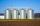# Concentration

How many grams of 65% and 50% acid is to be mixed to give 240 g acid with a concentration of 60%?

Result

a =  160
b =  80

#### Solution:

a+b= 240
0.65a+0.50b = 0.60*240

a+b = 240
0.65a+0.5b = 144

a = 160
b = 80

Calculated by our linear equations calculator.

Leave us a comment of example and its solution (i.e. if it is still somewhat unclear...):

Showing 0 comments:Be the first to comment!#### To solve this example are needed these knowledge from mathematics:

Our percentage calculator will help you quickly calculate various typical tasks with percentages. Do you have a linear equation or system of equations and looking for its solution? Or do you have quadratic equation?

## Next similar examples:

1. MushroomsMushrooms lose 90% by weight drying. How many fresh mushrooms are needed for 5 kg of dried mushrooms?
2. WarehousesIn the three warehouses, a total of 70 tons of grain was stored. In the second warehouse was stored 8.5t less and in the third 3.5t more than in the first. How many tons of grain was stored in each warehouse?
3. PotatoesFor three days the store sold 1400 kg of potatoes. The first day they sold 100 kilograms of potatoes less than the second day, the third-day three-fifths of what they sold the first day. How many kgs of potatoes sold every day?
4. EmployeesThere are 1116 people working in three factory halls. In the first one, there are 18% more than the third, and 60 persons more than the second. How many employees work in individual halls?
5. Company and employeesThere are 370 employees in the company - women are 15% less than men. How many men work in the company?
6. ChildrenThe group has 42 children. There are 4 more boys than girls. How many boys and girls are in the group?
7. Three workshopsThere are 2743 people working in three workshops. In the second workshop works 140 people more than in the first and in third works 4.2 times more than the second one. How many people work in each workshop?
8. Three unknownsSolve the system of linear equations with three unknowns: A + B + C = 14 B - A - C = 4 2A - B + C = 0
9. Linsys2Solve two equations with two unknowns: 400x+120y=147.2 350x+200y=144
10. Three friendsDanica, Lenka and Dalibor have altogether 96 kg. Lenka weighs 75% more than Dalibor and Danica weighs 6 kg more than Dalibor. Determine the weight of Danice, Lenka and Dalibor.
11. Alcohol mixingHow much 55% alcohol we must pour into 1500 g 80% alcohol to form a 60% alcohol? How much 60% alcohol created?
12. Vegan shopTo the shops brought together 23.2 kg of goods, fruits, vegetables and nuts. fruit was 4.7 kg more than vegetables, nuts was 1.5 kg less than the fruit. Determine the amount of fruits, vegetables and nuts.
13. Profit gainIf 5% more is gained by selling an article for Rs. 350 than by selling it for Rs. 340, the cost of the article is:
14. Elimination methodSolve system of linear equations by elimination method: 5/2x + 3/5y= 4/15 1/2x + 2/5y= 2/15
15. LegsCancer has 5 pairs of legs. The insect has 6 legs. 60 animals have a total of 500 legs. How much more are cancers than insects?
16. Ball gameRichard, Denis and Denise together scored 932 goals. Denis scored 4 goals over Denise but Denis scored 24 goals less than Richard. Determine the number of goals for each player.
17. Theorem proveWe want to prove the sentense: If the natural number n is divisible by six, then n is divisible by three. From what assumption we started?